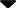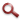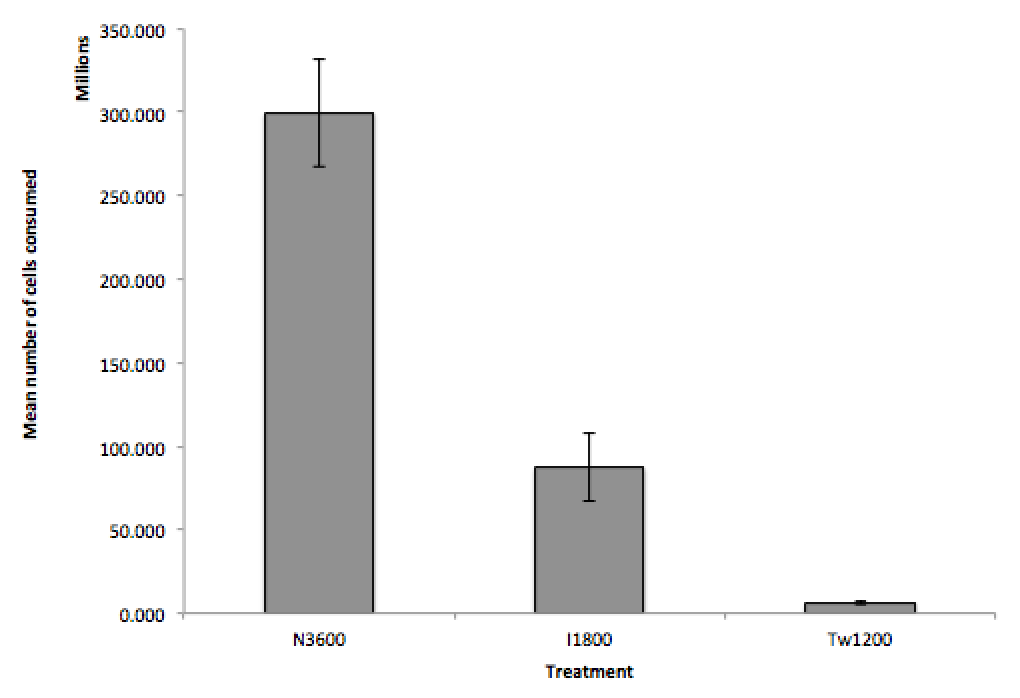Site WebYou are here:

## Student Project FiguresFigure 5 Figure 5 - Mean number of cells consumed in each algal treatment: Nannochloropsis sp. (N3600, n=13, mean=300±32million cells), T-Iso sp. (I1800, n=14, mean=88±20million cells) and Thalassiosira sp. (Tw1200, n=13, mean=6±0.8million cells). Error bars display ±1 standard error. The change in between the three treatments was significantly different (ANOVA: F2,40=46.43, P<0.001). Figure 4 Figure 5 Figure 6